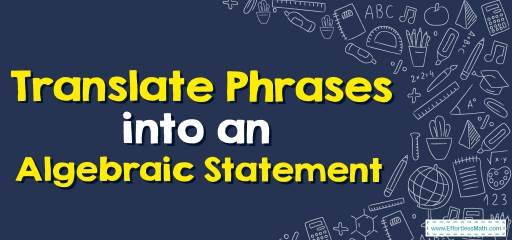# How to Translate Phrases into an Algebraic Statement? (+FREE Worksheet!)

To solve some algebra problems, sometimes you need to translate phrases into algebraic statements then solve the problem.## Step by step guide to translating phrases into an algebraic Statement

Translating key words and phrases into algebraic expressions:

• Addition: plus, more than, the sum of, etc.
• Subtraction: minus, less than, decreased, etc.
• Multiplication: times, product, multiplied, etc.
• Division: quotient, divided, ratio, etc.

The Absolute Best Book to Ace Pre-Algebra

### Translate Phrases into an Algebraic Statement – Example 1:

Write an algebraic expression for this phrase “$$12$$ times the sum of $$5$$ and $$x$$”.

Solution:

Sum of $$5$$ and $$x: 5 \ + \ x$$. Times means multiplication. Then: $$12 \ × \ (5 \ + \ x)$$

### Translate Phrases into an Algebraic Statement – Example 2:

Write an algebraic expression for this phrase. “Nine more than a number is $$18$$”

Solution:

More than mean plus, a number $$=x$$
Then: $$9 \ + \ x=18$$

### Translate Phrases into an Algebraic Statement – Example 3:

Write an algebraic expression for this phrase. “Eight more than a number is $$20$$”

Solution:

More than mean plus, a number $$=x$$
Then: $$8+x=20$$

### Translate Phrases into an Algebraic Statement – Example 4:

Write an algebraic expression for this phrase. “$$5$$ times the sum of $$8$$ and $$x$$”

Solution:

Sum of $$8$$ and $$x: 8+x$$. Times means multiplication. Then: $$5×(8 +x)$$

## Exercises for Translating Phrases into an Algebraic Statement

A Perfect Practice Workbook for Algebra I

### Write an algebraic expression for each phrase.

• The number increased by forty–two.
• The sum of fifteen and a number
• The difference between fifty–six and a number.
• The quotient of thirty and a number.
• Twice a number decreased by $$25$$.
• Four times the sum of a number and $$– 12$$.

• $$\color{blue}{x + 42}$$
• $$\color{blue}{15 + x}$$
• $$\color{blue}{56 – x}$$
• $$\color{blue}{\frac {30} {x}}$$
• $$\color{blue}{2x – 25}$$
• $$\color{blue}{4(x + (–12))}$$

Best Algebra Prep Resource

### What people say about "How to Translate Phrases into an Algebraic Statement? (+FREE Worksheet!) - Effortless Math: We Help Students Learn to LOVE Mathematics"?

No one replied yet.

X
45% OFF

Limited time only!

Save Over 45%

SAVE $40 It was$89.99 now it is \$49.99